## Monday, June 25, 2012

### On Run Distributions, pt. 4: Tango Distribution

At this point in the series, I need to take a detour from discussing run per game distributions and get a little more fundamental--runs per inning distributions. Thankfully, this will not involve any painful attempts to develop a model, as a good one already exists: the Tango Distribution.

In order to implement the zero-modified negative binomial model I’ve discussed, I need a way to estimate the probability of zero runs being scored in a game. While converting a runs per inning distribution to a runs per game distribution involves a mess of combination math, estimating the probability of a shutout from an inning distribution is much simpler. Theoretically, the probability of a shutout should be the probability of failing to score in a single inning to the ninth power (that is, the probability of failing to score in nine consecutive innings).

Of course, this requires making some assumptions, most notably that there are nine innings per game (in fact, if both teams fail to score, then in at least 50% of such games a team will fail to score in the first nine innings and still not get whitewashed for the game) and that the run distribution in each inning is independent.

The Tango Distribution uses Runs/Inning (RI) as one parameter, and a control value c as the other. c is set equal to .767 if looking at one team independently and .852 for teams in a head-to-head game. Then:

a = c*RI^2
f(0) = RI/(RI + a)
d = 1 - c*f(0)
f(1) = (1 - f(0))*(1 - d)
f(k) = f(k - 1)*d for k >= 2

I’ll assume that RI = (R/G)/9, so if we have a team that averages 4.4558 R/G, their RI = .4951.

a = .767*.4951^2 = .188
f(0) = .4951/(.4951 + .188) = .7248
probability(0 runs in game) = p(0) = f(0)^9 = .7248^9 = .0552

Our empirical probability of a shutout for teams averaging 4.4558 R/G was .0578.

We will use the Tango Distribution to estimate the parameter z for the Enby distribution. I’ll restate the formula here, omitting the superfluous formulas for the probabilities of scoring in the inning:

z = (RI/(RI + c*RI^2))^9 where default is c = .767

As I mentioned earlier, Ben Vollmayr-Lee demonstrated how to use the Tango Distribution to estimate the per game run distribution, assuming that runs are independent across innings. His explanation is available here (zip link).

This provides us an alternative means of estimating the runs per game distribution. It’s really not important to me at this whether the converted Tango Distribution or the Enby distribution does better, as the latter is easier to implement and I’ve surely not been able to optimize it. In any event, the Tango Distribution is a very valuable tool to have as well, certainly for estimating the runs per inning distribution but also for the runs per game distribution.

I have a spreadsheet which implements Vollmayr-Lee’s approach, and used it to generate estimated runs per game distributions for the three samples I’ve referenced throughout the series. I’ll give you the graphs here as I did for the Enby distribution. First, for all teams 1981-1996: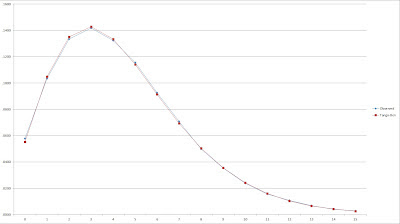On the eyeball test, it certainly does seem as if the Tango-Ben method is a better fit than the Enby distribution, especially when we consider that the latter has a number of artificial advantages in this test. Here are the 25 lowest scoring teams:This doesn’t look as good, but remember the sample size caveat and it still looks a little better than the Enby distribution. For the 25 highest scoring teams:Pretty good, again appearing to be a better fit than Enby. Again, I want to stress that I don’t think Enby is any kind of silver bullet; its potential value is due to its being a distribution that returns a direct result in terms of runs per game and is a well-known distribution.

## Monday, June 18, 2012

### On Run Distributions, pt. 3: Zero Modification

It appears that the negative binomial distribution provides a decent starting point for estimating the per game run distribution for typical major league teams. However, even a quick glance at the results suggests that it underestimates the probability of a team being shutout, and overestimates the probability of scoring a moderate number of runs (2-4).

While what I’m going to propose in this piece may appear to just be a crude fudge factor, but in fact distributions with modifications at zero are in use (Google “zero modified distributions” or something similar if you have to see for yourself). Modifying the negative binomial distribution to allow more probability to be placed at the point 0 runs will obviously improve our estimate of that probability, but should also result in more accurate estimates across the board.

I’ll let the variable a represent the probability of zero using the negative binomial distribution without modification, while z will represent the new probability that we wish to place on the zero point (in effect, it is now a third parameter along with r and B). So:

a = (1 + B)^(-r)

To calculate the probability of j runs (where j >=1) in this new distribution, all we do is assume that the share of non-zero probability assigned to each j stays the same--the only difference is that there is now a different amount of non-zero probability available. Recall that:

q(k) = (r)(r + 1)(r + 2)(r + 3)...(r + k - 1)*B^k/(k!*(1 + B)^(r + k)) for k >=1

So:

p(k) = z for k = 0
p(k) = (1 - z)*q(k)/(1 - a) for k >=1

In order to demonstrate this, I’m going to look at the three groupings of 1981-1996 teams I used last time: all teams and the 25 lowest and highest scoring teams. In this case I will set z equal to the actual observed frequency of scoring zero runs. Obviously, this is cheating if we are trying to establish the accuracy of the model and not helpful in cases in which we don’t know the empirical probability of zero runs. Again, though, I’m doing it this way as a quick check of whether or not we’re wasting our time. If you can’t make material gains in the accuracy with a known value, good luck trying to do so while estimating that value.

For the average teams, z = .0578 and a was previously calculated to be .0495. We previously estimated that the probability of scoring 3 runs was .1468, so the new estimated probability of scoring 3 runs is:

p(3) = (1 - .0578)*.1468/(1 - .0495) = .1455

Here’s a chart: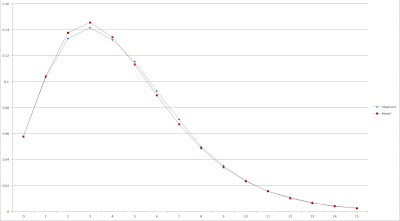The model is still guessing too high on the probability of scoring a moderate number of runs, but at least now we no longer have one point which is an obvious problem. Going back to the X^2 statistic I discussed previously, the X^2 (excluding zero runs, since we’ve set this up in such a manner that the error at the point is zero by definition) for the zero-modified model is 63 compared to 68 for the unmodified distribution. That’s a slight gain in accuracy; in order for it to be worth the extra effort, the gain in better estimating shutouts has to be worth the extra work. For the lowest and highest scoring teams, the gains are similar.

Unfortunately, the zero-modification has a side effect which reduces the accuracy and (more importantly) destroys a fundamental property that our run distribution model must adhere to. I’d posit there are two such fundamental properties--obviously there are other aspects to consider, but these two are non-negotiable:

1. The model must return a total probability of 1. That is, p(0) + p(1) + p(2) + … + p (infinity) = 1. This property is not affected by the zero-modification.

2. The model must return the correct average runs scored. That is, 0*p(0) + 1*p(1) + 2*p(2) + 3*p(3) + ... = R/G. This property is not preserved by the zero-modified distribution.

Remember that when I fit the parameters initially, I used the method of moments, which relies on the mean of the distribution. The mean of a negative binomial distribution is r*B, and by using the method of moments I ensured that the mean and variance of the fitted distribution would equal the mean and variance of the sample.

In the literature on zero-modified distributions (see here for an example) one can find formulas for the mean and variance of the modified distributions. Ideally, we could just use the method of moments in conjunction with these formulas and fit the distributions properly. Unfortunately, that’s not possible:

mean = (1 - z)*r*B/(1 - (1 + B)^(-r))
variance = (1 - z)*rB*((1 + B) - (1 + B + r*B)*(1 + B)^(-r))/((1 - (1 + B)^(-r))^2 + z*(1 - z)*((1 - z)*r*B/(1 - (1 + B)^(-r)))^2

In fact, even the formula for the mean makes it impossible to algebraically force the mean of the modified distribution to equal the sample mean. Even if you hold either r or B constant, there’s no simple algebraic solution.

This complicates the decision between the modified and standard distribution considerably; if we use the standard distribution, we know that we’ll underestimate the probability of a shutout for all manner of teams. If we use the modified distribution, we won’t retain the same mean and variance as observed in the sample. What to do?

From my limited testing, it appears as if the least disruptive approach is to set the value of r such that the mean is equal to the sample mean. By least disruptive, I mean that the variance of the modeled distribution is closer to the variance of the sample when r is changed rather than B. For the sample of all teams, the revised value of r necessary is approximately 4.09, which results in a variance of 9.5663 (the sample variance is 9.3603). Changing B would result in a new B value of approximately 1.112 with a variance of 9.61.

Ideally both r and B would be selected in such a way that would maximize accuracy, but that is beyond my capabilities given the complexity of the model and three parameters in play. So I’ll have to settle for a crude solution, which will be to vary r.

Since there is no algebraic solution to calculate the necessary value of r, this will require an ad hoc approach. For those that are interested, I have gone through the trouble of figuring the parameters for common R/G levels and will present those at the end of this series.

Here is a graph of the modified distribution:We’re still underestimating the likelihood of 2-4 runs and overestimating the likelihood of 5-7 runs, but I’m unaware of any other discrete distribution that comes close to this level of accuracy.

I’m getting a little tired of writing "negative binomial" and "modified", and so I will slap a cutesy name on this method. I’m not going to name it after myself, of course (for the record, I didn’t push the use of “Pythagenpat”, and this is only a new application of a common probability distribution discovered centuries ago). Until someone else decides they want to use this method and give it a better name, I’ll go with the Enby Distribution. Abbreviate “negative binomial” to “NB” and try spelling it as a word, and you get Enby.

## Monday, June 11, 2012

### On Run Distributions, pt. 2: Negative Binomial

There does exist a common discrete distribution that can be useful in modeling the per game run distribution. It doesn’t necessarily seem like it should work, since the questions that the distribution is typically employed to answer (at least in the sort of non-expert presentations most people are exposed to) doesn’t have an obvious connection with expected runs scored in baseball. Is it a perfect fit? Of course not; there are no perfect models anyway, but even granting that, it has flaws. Would it work in extreme circumstances? I don’t really know; over the next several articles I’ll take a stab at answering these questions, but obviously I have my limits as an analyst.

I did a couple of internet searches to try to see if there were any published papers or articles using the Negative Binomial distribution to model runs scored. There were a few that seemed to mention it, and there was an article about cricket scoring, but I didn’t see anything using it for the exact purpose I am. That does not mean none exist.

In any event, I know that I am not the first person to use the distribution to model runs per game, because I was sent an unpublished paper about it several years ago by Phil Melita. Melita sent it to me since he had cited Pythagenpat in the article, and we traded a couple emails about it. It was never published in By the Numbers, though, and I’m not sure why. In any event, Melita didn’t present his work in the same manner that I am here, but it was clearly along the same lines and I can take no credit for any originality here. I hadn’t thought about it in a few years, but as soon as I tried the negative binomial, I remembered Melita’s article.

To be honest, the negative binomial is not one of my favorite distributions. From my educational experiences, it is associated with two things in my mind: 1) the nice, simple Geometric distribution (a special case of negative binomial) run amuck and 2) Poisson-Gamma compound processes. It does have other obvious baseball uses, but it’s just not as intuitive to me as Poisson, Geometric, or Binomial.

The way the negative binomial distribution is usually presented masks its ability to deal with fractional parameters, hidden by the use of factorials and the aversion of everyone but mathematicians to gamma functions. And the problems to which the negative binomial is usually applied are along the lines of “What is the probability of 5 successes before the eighth failure?”, which further reduces the likelihood that people will consider it as a model for run scoring. However, the pmf for the negative binomial can be written thusly:

p(k) = (1 + B)^(-r) for k = 0
p(k) = (r)(r + 1)(r + 2)(r + 3)…(r + k - 1)*B^k/(k!*(1 + B)^(r + k)) for k >=1

where r and B are the parameters of the distribution and k is the point at which we are calculating the probability.

Of course, in order to actually use this or any other distribution, we need to be able to define the parameters. Everything that follows in this series of posts needs to be prefaced by this caveat--I am not a statistician or a probability expert. Compared to the average person, I have a lot of knowledge through my own curiosity and my studies/profession. Compared to the average person who dabbles in sabermetrics, though, my knowledge doesn’t stand out, and obviously I am a kindergartner compared to the experts in these fields.

So here I will take the easy step of fitting the parameters of this distribution using the method of moments. There may well be a better, more precise method out there (maximum likelihood estimation always comes to mind, but is a nightmare for negative binomial). In any event, the most important simple properties of any distribution are its mean and variance. For negative binomial, these are computed as follows:

mean (u) = r*B
variance(v) = r*B*(1 + B)

The method of moments uses the first two central moments (mean and variance to estimate the parameters). If we take the ratio of v to u, we can estimate B:

v/u = r*B*(1 + B)/(r*B) = 1 + B
Ergo B =v/u - 1 and r = u/B = u^2/(v - u).

For 1981-96, the average runs scored per game was 4.4558 with a variance of 9.3603, which leads to an estimate that B = 9.3603/4.4558 - 1 = 1.1007 and r = 4.4558/1.1007 = 4.0480.

Given those parameters, here are the estimates for the first few run totals:

p(0 runs) = (1 + B)^(-r) = (1 + 1.1007)^(-4.048) = .0496
p(1 run) = r*B^k/(k!*(1 + B)^(r + k)) = 4.048*1.1007/(1!*(1 + 1.1007)^(4.408 + 1)) = .1051
p(2 runs) = r*(r + 1)*B^k/(k!*(1 + B)^(r + k)) = 4.408*(4.408 + 1)*1.1007^2/(2!*(1 + 1.1007)^(4.408 + 2)) = .1390
p(3 runs) = r*(r + 1)*(r + 2)*B^k/(k!*(1 + B)^(r + k)) = 4.408*(4.408 + 1)*(4.408 + 2)*1.1007^3/(3!*(1 + 1.1007)^(4.408 + 3)) = .1468

The r*(r + 1)*(r + 2)*(r + 3)*(r + 4)*... formula gets annoying, but with a spreadsheet it is easy to implement. Here is the complete runout (to 16 runs), along with a graph comparing the estimate probabilities to the observed frequencies: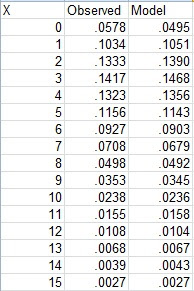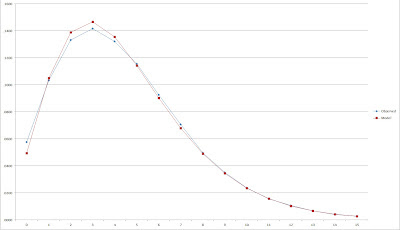When I look at that graph, I see a pretty decent fit, one that makes me believe that the negative binomial distribution may have some usefulness in estimating run distributions. Is it perfect? No; as you can see, it is noticeably low on shutouts (an issue that I will go into detail about in the next post), and it’s too high for some of the other common scores (2-4). Using the X^2 test statistic described in the previous post, this scores a 176, which is easily better than the other crude models I considered.

Of course, I’ve only dealt with scoring at normal levels. In order to estimate run distributions for other scoring levels, we’ll have to change the parameters. The mean number of runs per game is always known, but the variance is a quantity that we can only know if we have prior knowledge of the empirical run distribution. Since both r and B are dependent on both the mean and variance, they will be constantly changing when the mean and variance of the distribution we are fitting to changes.

Before worrying about how to tackle that problem, we first need to make sure it would be worth attempting to adapt to non-average situations. I will consider the top and bottom 5% of teams by R/G for the data in Davenport’s spreadsheet--the 25 lowest and highest scoring teams of 1981-2006. The bottom 25 teams averaged 3.49 R/G with a variance of 6.6206, which produces estimates of B = .897 and r = 3.891. The results:This doesn’t look as nice, but it still appears as if the model does a decent job. Remember that the sample size is not that large (25 teams is a smaller sample than a full major league season), so there’s the possibility of some random fluctuation. Again, the point here is not to perform a rigorous test, but rather to make sure the model isn’t completely out in left field.

The 25 highest scoring teams averaged 5.7 R/G with a variance of 12.4 for B = 1.175 and r = 4.849: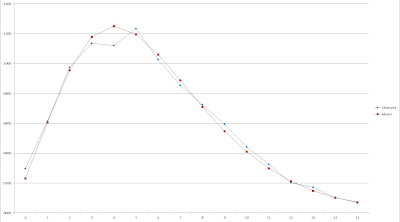Not bad. Next time, I’ll discuss a workaround for the underestimation at zero runs (albeit one that introduces a new stumbling block).

## Monday, June 04, 2012

### On Run Distributions, pt.1: Introduction

Let’s suppose that you were going to conjure up a model that fit the distribution of team runs per game. What properties would you like it to exhibit (ignoring for the moment whether a model with such properties is actually feasible)?

I would suggest the following:

1. It should be discrete. Runs scored in a game is a discrete quantity. There’s no such thing as a fractional number of runs scored. There’s nothing wrong with suggesting that a certain combination of events will generally create, say, 4.35 runs, but that 4.35 runs represents a weighted average of all the times that combination produces 0, 1, 2, 3, 4, 5, …, runs. The distribution function should reflect those probabilities.

2. It should be a standard, well-known probability distribution.

3. It should be accurate, and adaptable across a wide range of scoring contexts. This is the most important, but also should go without saying.

4. If possible, it should be simple. I am not suggesting trading accuracy or theoretical purity for simplicity, but this is a wish list.

Again, that is a wish list. In the real world, you have to model reality. That might mean accepting a continuous distribution as a model of a discrete function, or having a method that is useful in normal contexts but has some structural deficiencies that limits its utility at the extremes.

If we want to find a distribution that meets some of the criteria on the list, we have to start with discrete distributions. I would assume that the first such distribution that comes to mind for most people with any background in probability or statistics is the Poisson distribution. The Poisson distribution is simple, adaptable, and has a cool name and a cool Greek letter for a parameter. Unfortunately, it doesn’t work as a model of runs scored in baseball, despite some noble efforts to make it so.

Poisson does work fairly well (at least to the extent of my knowledge, which is limited) as a model of soccer and hockey scoring. Given that it is often used for situations in which events are expected to occur over a certain period of time (or area and other physical intervals), it makes intuitive sense that it wouldn’t fit baseball run scoring, since time is not a factor. A Poisson process should be able to be extended to time periods other than the basis--that is, if your mean is 3 goals per game for hockey, you should be able to use the distribution to estimate goals per period (with mean 1) or goals per ten games (with mean 30), or goals per 3714 games (with mean 11142).

This property obviously does not carry over to baseball due to the nature of the game. One could picture it working for converting between innings and games, but once you get to the inning level and start splitting into outs, the pattern of runs scored is not the same independent of the number of outs in the inning (i.e. more runs are scored with two out than zero out).

As you can see in the chart in the Clay Davenport article I linked above, the Poisson distribution severely underestimates the proportion of shutouts. This is an issue that can be sidestepped by using a modified value at zero, but the slope at non-zero runs is not close enough to reality to salvage a Poisson approach, and the relationship of the mean of runs scored in relation to the variance is not a match for Poisson.

In lieu of a simple distribution, those examining baseball scoring have had to be creative. The three-parameter Weibull distribution (a continuous distribution) has been used with good results by Dr. Steven Miller and Sal Baxamusa to model runs scored. The Tango Distribution, which models runs scored per inning, can be used to estimate the per game distribution with the use of some combination math published by Ben Vollmayr-Lee (zip link) (as an aside, one of the topics in statistics that confuses me the most is combinations and permutations. Sure, I understand why 9! gives you the number of possible batting orders for nine players, and I understand the binomial coefficient, but start asking more detailed questions about pulling colored balls out of urns with and without replacement and I started to wish that I was studying liberal arts.)

With the recent publication of Bill James’ old Baseball Analyst newsletters, I was able to read Dallas Adams’ article in the June 1982 issue in which he uses the following functions to estimate scoring distribution as a function of R/G:

When N is 5 runs or fewer: P(N) = A + B*N + C^2*N

where A = .38498 - .10839 (R/G) + .0080055(R/G)^2
B = .0010306 + .024139 (R/G) - .002943(R/G)^2
C = -.01879 + .002514(R/G) - .00001506(R/G)^2

When N is 6 runs or more: P(N) = D(E^N)
where D = 6.6210 - 2.4965(R/G) + .27518(R/G)^2
E = .058479 + .24022(R/G) - .022291(R/G)^2

That’s the kind of thing I’d like to avoid if at all possible, no offense to Mr. Adams and the others who have made the effort to work on this problem.

Let me close out this post by offering a quick look at the Adams formula and the failures of Poisson, while introducing a set of data that I’ll use to fit a model in the next installment. I have a spreadsheet (I believe it was put together by Clay Davenport or Keith Woolner, but I can’t find the original link) with the runs per game for each major league team 1981-96. The aggregate distribution for these teams was:The easiest way to fit a Poisson model to this data is to use the maximum likelihood estimate that the parameter h is equal to the sample mean (this is also equivalent to the method of moments estimate in this case). In this case the mean R/G is 4.456 and the variance is 9.36 (those of you familiar with Poisson can already see this is not going to end well as a Poisson distribution has a mean equal to its variance).

The probability mass function for Poisson is:
P(x) = e^(-h)*h^x/x!

For example, the estimated probability of scoring 3 runs is e^(-4.456)*4.456^3/3! = .1712. The empirical probability in this case is ,1417, and this is one of the more accurate points:As you can see, the Poisson model bunches too much probability around the mean, leaving estimates of high scoring games too low and estimates of low scoring games way too low.

I will also look at Adams’ formula just for kicks. The constants for 4.456 R/G are A = .0610, B = .0502, C = -.0079, D = .9606, E = .6863, yielding these estimated probabilities: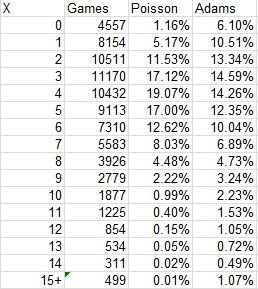Adams better matches the actual distribution for normal amounts of runs scored, but overestimates the likelihood of extremely high run output. Since he uses formulas that don’t tie to a true probability distribution which adheres to the requirements thereof (most importantly that the sum of all probabilities is one), the estimated probabilities total to 1.03 and runs per game to 4.61.

My point is not to pick on a thirty-year old formula from a pioneering sabermetrician--the fact that luminaries such as Adams and Davenport have not been able to come up with a satisfying approach to this problem serves to illustrate its difficulty, and hopefully to cause you not to judge too harshly the method that I offer in the next post as flawed but useful in certain circumstances.

Before closing this post, let me provide a crude measure of the relative accuracy of the models. Basically, it is the chi-square test statistic. For each number of runs scored (0, 1, 2, etc.), calculate (Expected games with n runs - actual games with n runs)/Expected^2. Here, I will group all games of 15+ runs together, which could be a problem if we encounter a distribution that overpredicts extremely high scores.

I will be doing this test (which I’ll call X^2) without any of the trappings of an actual chi-square test: degrees of freedom, critical values, and the like. The point is not to perform a real significance test--it's to get a quick idea of how these models stack up against one another. I will at various points in this series be testing the model with the same data that was used to fit its parameters--obviously a no-no if you want an unbiased test. However, the first basic test for a model is whether or not it can describe the data it was fitted with. If it can’t do that, there’s absolutely no point in worrying about how it handle out of sample data. None of the models I’m going to be offering up in this series are clearly good enough to move on to that next step.

So, for the Poisson, Adams, and modified Adams (I rescaled so that the total probability would be equal to one) distributions, here are the X^2 values for the model versus the 1981-96 data:
Poisson 91996